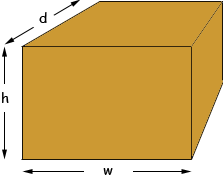I have word problem. Please help. I need to find the dimensions of a rectangular box. They ask for the areas of the top, side and end. If those areas are 120 square units, 96 square units, and 80 square units respectively, what are the dimensions of the box? thanks for your help!! Ben Hi Ben. Let's draw a box and label the height h, the width w and the depth d.Each of the areas is a rectangle and you know that you multiply the two dimensions of a rectangle together to get the area. This means in our diagram, The top is: 120 = wd , The side is: 96 = hd , and the end is: 80 = wh. Look what happens when we multiply the first two together and divide by the last:    (wd)(hd)       12096 ----------------------- = -------------           wh                    80 Now if we simplify, we can see that the w's and the h's cancel out, leaving an equation with only one variable in it! You should be able to calculate the depth and once you know that, you can use the areas to figure out the other two dimensions. I hope this helps! Stephen La Rocque>